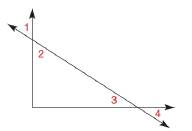Chapter 1.6, Problem 12E### Elementary Geometry for College St...

6th Edition
Daniel C. Alexander + 1 other
ISBN: 9781285195698

#### Solutions

Chapter
Section### Elementary Geometry for College St...

6th Edition
Daniel C. Alexander + 1 other
ISBN: 9781285195698
Textbook Problem
1 views

# In Exercise 11 and 12, provide the missing statements and reasons. Given: ∠ 1 ≅ ∠ 2 ; ∠ 3 ≅ ∠ 4 ∠ s 2 and 3 are complementary Prove: ∠ s 1 and 4 are complementary PROOF Statements Reasons 1. ∠ 1 ≅ ∠ 3 and ∠ 3 ≅ ∠ 4 1. ? 2. ? and ? 2. If two ∠ s are ≅ , then their measures are equal. 3. ∠ s 2 and 3 are complementary 3. ? 4. ? 4. The sum of the measures of complementary ∠ s is 90 5. m ∠ 1 + m ∠ 4 = 90 5. ? 6. ? 6. If the sum of the measures of two angles is 90 , then the angles are complementaryTo determine

To find:

The missing statements and reasons.

Explanation

Given:

12

34

s 2 and 3 are complementary

The given figure is,

Figure (1)

And,

The given table is shown below.

 PROOF Statements Reasons 1. ∠1≅∠2 and ∠3≅∠4 1. ? 2. ? and ? 2. If two ∠s are ≅, then their measures are equal. 3. ∠s 2 and 3 are complementary 3. ? 4. ? 4. The sum of the measures of complementary ∠s is 90 5. m∠1+m∠4=90 5. ? 6. ? 6. If the sum of the measures of two angles is 90, then the angles are complementary

Approach:

1. The perpendicular lines are two lines that meet to form congruent adjacent angles.

2. If two lines are perpendicular, then they meet to form right angles.

3. If two angles are congruent, their measures are equal.

4. The symmetric property for the congruence of angles is satisfied, If 12, then 21.

5. If the sum of the measures of two angles is 90, then the angles are complementary.

Calculation:

The reason for the first statement of the proof is “Given”, so the first statement is given as,

12

And,

34

The reason for the second statement of the proof is, “If two s are , then their measures are equal”. So, use this property in above equations.

m1m2

And,

m3m4

So, the second statements is,

m1m2

And,

m3m4

The reason for the third statement of the proof is “Given”. So, the statement is s 2 and 3 are complementary

### Still sussing out bartleby?

Check out a sample textbook solution.

See a sample solution

#### The Solution to Your Study Problems

Bartleby provides explanations to thousands of textbook problems written by our experts, many with advanced degrees!

Get Started